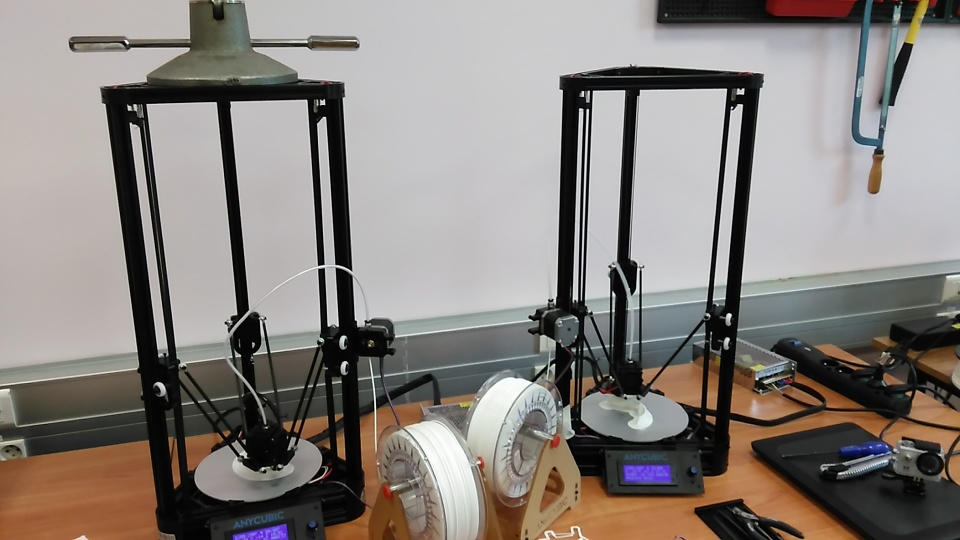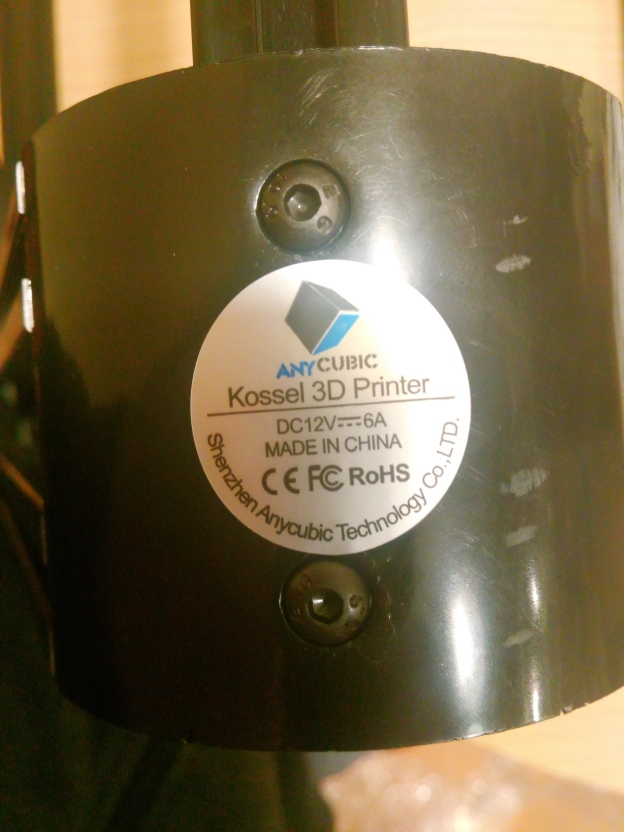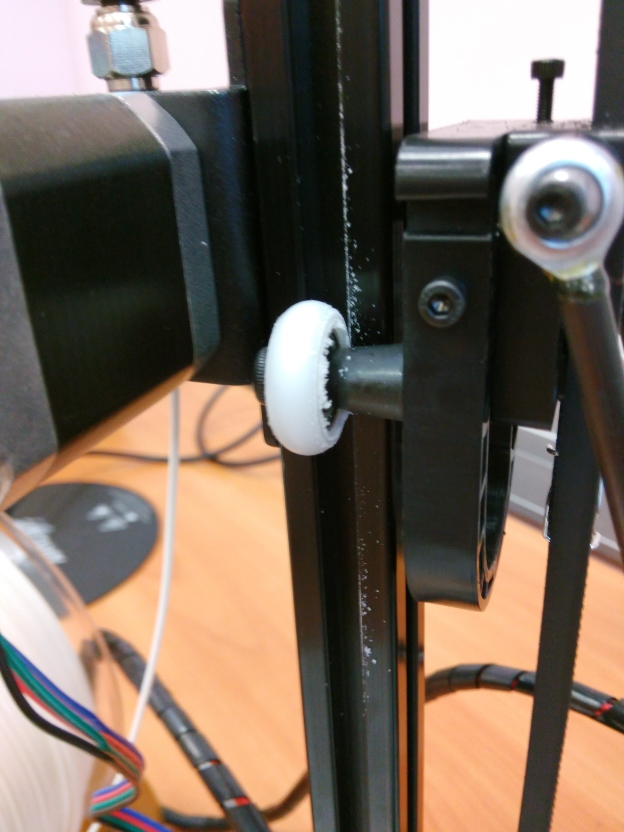# New robot for teaching… a 3D delta printerSo, lately during the summer I wanted to have some new robots for the students to work on. You know, some motors, couple of sensors, built-in Arduino-like electronics, that kinda stuff. And after about a week of searching I found a perfect robot … a 3D printer.

So, my new robot for students to destroy is the delta-style chinese 3D printer. More precisely, Anycubic Kossel pulley-based printer. And as a bonus, it even prints. Quite well, to be honest, at least with PLA.

After ordering 6 of them (4 for students, 1 for my tests, 1 for spare parts), DHL delivered 6 nice little boxes. The printer comes disassembled and it takes around 5h per each to get it to a working state.

The main controller is a Trigorilla board, an Arduino Mega 2560 in disguise… well, actually without any disguise at all, because in order to update the firmware you launch the Arduino IDE and upload the Marlin to it. So it’s perfectly hackable. Good.I chose not to use the heated bed, because you should power it with 240W 12V power supply that has no protection case at all – the 230V connections are at the front, and as I know my students – at least one would touch it by accident, especially since they are electrical engineers. So, no heated bed for me, the mainboard has a barrel connector which nicely fits my safe 60W power bricks that I have plenty of in a lab. The version I ordered have pulleys instead of linear slides (hiwin-clones) because it way cheaper and I could order one more printer for the same cost. The rollers will wear out pretty soon, but I will solve it with 3D printed recirculating carriages when the time comes.As per sensors we get 3 switches as endstops + free pins for 3 more and 1 temperature sensor at the hotend. This is enough for basic control. The only downside is that we will be forced to run motors in an open-loop, with step counting.

First, it’s good to know how the board looks like… oh, not much info? I tried at first to search on Google Images for RAMPS pinout, but the best was the info about a shield for arduino mega:
http://reprap.org/mediawiki/images/3/3f/Arduinomegapololushieldschematic.png
… and I was not amazed. Well, it’s a RAMPS variant, so the file pins_RAMPS.h in Marlin is easier to decipher
https://github.com/MarlinFirmware/Marlin/blob/1.1.x/Marlin/pins_RAMPS.h

I will copy the corresponding defines as needed. Our first example program will be reading a state of one of the switches, for example on the x-axis

```#define X_MAX_PIN           2

int buttonX = 0;

void setup()
{
pinMode(X_MAX_PIN, INPUT);
digitalWrite(X_MAX_PIN, HIGH);

Serial.begin(57600);
Serial.println("oh, hello");
}

void loop()
{
Serial.println(buttonX);
delay(10);
}
```

Similar thing can be done for the rest:

```#define X_MIN_PIN           3
#define X_MAX_PIN           2
#define Y_MIN_PIN          14
#define Y_MAX_PIN          15
#define Z_MIN_PIN          18
#define Z_MAX_PIN          19
```

Now for a single stepper:

```#define X_MAX_PIN           2

#define X_STEP_PIN         54
#define X_DIR_PIN          55
#define X_ENABLE_PIN       38

int buttonX = 0;

void setup()
{
pinMode(X_MAX_PIN, INPUT);
digitalWrite(X_MAX_PIN, HIGH);

pinMode(X_STEP_PIN, OUTPUT);
pinMode(X_DIR_PIN, OUTPUT);
pinMode(X_ENABLE_PIN, OUTPUT);
digitalWrite(X_DIR_PIN, LOW);
digitalWrite(X_ENABLE_PIN, LOW);
}

void loop()
{
if (buttonX == 0) {
digitalWrite(X_STEP_PIN, HIGH);
delay(1);
digitalWrite(X_STEP_PIN, LOW);
delay(1);
}
}
```

You don’t need X_CS_PIN, unless you go with a SPI-based stepper drivers (Trinamic). Now for all three axis, with a bit faster delayMicroseconds, and an ability to count steps:

```#define X_MIN_PIN           3
#define X_MAX_PIN           2
#define Y_MIN_PIN          14
#define Y_MAX_PIN          15
#define Z_MIN_PIN          18
#define Z_MAX_PIN          19

#define X_STEP_PIN         54
#define X_DIR_PIN          55
#define X_ENABLE_PIN       38

#define Y_STEP_PIN         60
#define Y_DIR_PIN          61
#define Y_ENABLE_PIN       56

#define Z_STEP_PIN         46
#define Z_DIR_PIN          48
#define Z_ENABLE_PIN       62

int buttonX = 0, buttonY = 0, buttonZ = 0;
int stepsX = 0, stepsY = 0, stepsZ = 0;

void setup()
{
pinMode(X_MAX_PIN, INPUT);
pinMode(Y_MAX_PIN, INPUT);
pinMode(Z_MAX_PIN, INPUT);
digitalWrite(X_MAX_PIN, HIGH);
digitalWrite(Y_MAX_PIN, HIGH);
digitalWrite(Z_MAX_PIN, HIGH);

pinMode(X_STEP_PIN, OUTPUT);
pinMode(X_DIR_PIN, OUTPUT);
pinMode(X_ENABLE_PIN, OUTPUT);
digitalWrite(X_DIR_PIN, LOW);
digitalWrite(X_ENABLE_PIN, LOW);

pinMode(Y_STEP_PIN, OUTPUT);
pinMode(Y_DIR_PIN, OUTPUT);
pinMode(Y_ENABLE_PIN, OUTPUT);
digitalWrite(Y_DIR_PIN, LOW);
digitalWrite(Y_ENABLE_PIN, LOW);

pinMode(Z_STEP_PIN, OUTPUT);
pinMode(Z_DIR_PIN, OUTPUT);
pinMode(Z_ENABLE_PIN, OUTPUT);
digitalWrite(Z_DIR_PIN, LOW);
digitalWrite(Z_ENABLE_PIN, LOW);

stepsX = 1000;
stepsY = 1000;
stepsZ = 1000;
}

void loop()
{
if (buttonX == 0 && stepsX > 0) {
digitalWrite(X_STEP_PIN, HIGH);
stepsX--;
}
if (buttonY == 0 && stepsY > 0) {
digitalWrite(Y_STEP_PIN, HIGH);
stepsY--;
}
if (buttonZ == 0 && stepsZ > 0) {
digitalWrite(Z_STEP_PIN, HIGH);
stepsZ--;
}
delay(1);
digitalWrite(X_STEP_PIN, LOW);
digitalWrite(Y_STEP_PIN, LOW);
digitalWrite(Z_STEP_PIN, LOW);
delay(1);
}
```

Now it’s a good time to introduce communication. There are a couple of methods to get the communication with our robot going, the first (and the worst) begin sending three numbers “250 100 300\n” as a number of steps to be taken by each axis and using parseInt() to capture them. Why bad? It’s not a protocol! Doing something like this:

```        char enter;
while( Serial.available() ) {
stepsX = Serial.parseInt();
stepsY = Serial.parseInt();
stepsZ = Serial.parseInt();
}
```

and expecting it to always work is just asking for trouble. It’s better to use the default route, with a character buffer and sscanf. The message will be something like “x 250 y 300 z 250\n”:

```        // global
char a;
int i = 0;
...
while(Serial.available()) {
if (a[i]!='\n') {
i++;
} else {
sscanf(a, "x %d y %d z %d\n", &stepsX, &stepsY, &stepsZ);
memset(a,0,sizeof(a));
i = 0;
}
}
```

Other weird options you may on some lonely day try to explore including using readString – but this comes with a burden of waiting in ms for the timeout (yes, it’s time based reading)

```        Serial.setTimeout(100);
...
String a;
while(Serial.available()) {
sscanf(a.c_str(), "x %d y %d z %d\n", &stepsX, &stepsY, &stepsZ);
}
```

or a hybrid design with an overloaded operator for String:

```        String a;
char b;
...
while(Serial.available()) {
a = a+b;
if (b=='\n') {
sscanf(a.c_str(), "x %d y %d z %d\n", &stepsX, &stepsY, &stepsZ);
a = String("");
}
}
```

I’m gonna go char[] route. Also, it would be nice to control the motors’ direction by sending negative numbers. The following should do the trick

```        if (stepsX < 0) {
dirX = 0;
digitalWrite(X_DIR_PIN, HIGH);
stepsX = -stepsX;
} else {
dirX = 1;
digitalWrite(X_DIR_PIN, LOW);
}
...
if (stepsX > 0) {
if ((buttonX == 0 && dirX == 1) || dirX == 0) {
digitalWrite(X_STEP_PIN, HIGH);
stepsX--;
}
}
```

Full program so far, this time with delayMicroseconds() for faster movement

```#define X_MIN_PIN           3
#define X_MAX_PIN           2
#define Y_MIN_PIN          14
#define Y_MAX_PIN          15
#define Z_MIN_PIN          18
#define Z_MAX_PIN          19

#define X_STEP_PIN         54
#define X_DIR_PIN          55
#define X_ENABLE_PIN       38

#define Y_STEP_PIN         60
#define Y_DIR_PIN          61
#define Y_ENABLE_PIN       56

#define Z_STEP_PIN         46
#define Z_DIR_PIN          48
#define Z_ENABLE_PIN       62

int buttonX = 0, buttonY = 0, buttonZ = 0;
int stepsX = 0, stepsY = 0, stepsZ = 0;
int dirX = 1, dirY = 1, dirZ = 1;

char message;
int i = 0;

void setup()
{
pinMode(X_MAX_PIN, INPUT);
pinMode(Y_MAX_PIN, INPUT);
pinMode(Z_MAX_PIN, INPUT);
digitalWrite(X_MAX_PIN, HIGH);
digitalWrite(Y_MAX_PIN, HIGH);
digitalWrite(Z_MAX_PIN, HIGH);

pinMode(X_STEP_PIN, OUTPUT);
pinMode(X_DIR_PIN, OUTPUT);
pinMode(X_ENABLE_PIN, OUTPUT);
digitalWrite(X_DIR_PIN, LOW);
digitalWrite(X_ENABLE_PIN, LOW);

pinMode(Y_STEP_PIN, OUTPUT);
pinMode(Y_DIR_PIN, OUTPUT);
pinMode(Y_ENABLE_PIN, OUTPUT);
digitalWrite(Y_DIR_PIN, LOW);
digitalWrite(Y_ENABLE_PIN, LOW);

pinMode(Z_STEP_PIN, OUTPUT);
pinMode(Z_DIR_PIN, OUTPUT);
pinMode(Z_ENABLE_PIN, OUTPUT);
digitalWrite(Z_DIR_PIN, LOW);
digitalWrite(Z_ENABLE_PIN, LOW);

Serial.begin(57600);
}

void loop()
{
while(Serial.available()) {
if (message[i]!='\n') {
i++;
} else {
sscanf(message, "x %d y %d z %d\n", &stepsX, &stepsY, &stepsZ);
memset(message,0,sizeof(message));
i = 0;
}

if (stepsX < 0) {
dirX = 0;
digitalWrite(X_DIR_PIN, HIGH);
stepsX = -stepsX;
} else {
dirX = 1;
digitalWrite(X_DIR_PIN, LOW);
}

if (stepsY < 0) {
dirY = 0;
digitalWrite(Y_DIR_PIN, HIGH);
stepsY = -stepsY;
} else {
dirY = 1;
digitalWrite(Y_DIR_PIN, LOW);
}

if (stepsZ < 0) {
dirZ = 0;
digitalWrite(Z_DIR_PIN, HIGH);
stepsZ = -stepsZ;
} else {
dirZ = 1;
digitalWrite(Z_DIR_PIN, LOW);
}
} // Serial.available()

if (stepsX > 0) {
if ((buttonX == 0 && dirX == 1) || dirX == 0) {
digitalWrite(X_STEP_PIN, HIGH);
stepsX--;
}
}
if (stepsY > 0) {
if ((buttonY == 0 && dirY == 1) || dirY == 0) {
digitalWrite(Y_STEP_PIN, HIGH);
stepsY--;
}
}
if (stepsZ > 0) {
if ((buttonZ == 0 && dirZ == 1) || dirZ == 0) {
digitalWrite(Z_STEP_PIN, HIGH);
stepsZ--;
}
}
delayMicroseconds(100);
digitalWrite(X_STEP_PIN, LOW);
digitalWrite(Y_STEP_PIN, LOW);
digitalWrite(Z_STEP_PIN, LOW);
delayMicroseconds(100);
}
```

That’s long! And repetitive – two main reasons to compact it into functions.

```#define X_MIN_PIN           3
#define X_MAX_PIN           2
#define Y_MIN_PIN          14
#define Y_MAX_PIN          15
#define Z_MIN_PIN          18
#define Z_MAX_PIN          19

#define X_STEP_PIN         54
#define X_DIR_PIN          55
#define X_ENABLE_PIN       38

#define Y_STEP_PIN         60
#define Y_DIR_PIN          61
#define Y_ENABLE_PIN       56

#define Z_STEP_PIN         46
#define Z_DIR_PIN          48
#define Z_ENABLE_PIN       62

byte buttonX = 0, buttonY = 0, buttonZ = 0;
int stepsX = 0, stepsY = 0, stepsZ = 0;
byte dirX = 1, dirY = 1, dirZ = 1;

char message;
int i = 0;

void set_pin(byte pin, byte mode, byte state)
{
pinMode(pin, mode);
digitalWrite(pin, state);
}

void setup()
{
set_pin(X_MAX_PIN, INPUT, HIGH);
set_pin(Y_MAX_PIN, INPUT, HIGH);
set_pin(Z_MAX_PIN, INPUT, HIGH);

set_pin(X_STEP_PIN, OUTPUT, LOW);
set_pin(X_DIR_PIN, OUTPUT, LOW);
set_pin(X_ENABLE_PIN, OUTPUT, LOW);

set_pin(Y_STEP_PIN, OUTPUT, LOW);
set_pin(Y_DIR_PIN, OUTPUT, LOW);
set_pin(Y_ENABLE_PIN, OUTPUT, LOW);

set_pin(Z_STEP_PIN, OUTPUT, LOW);
set_pin(Z_DIR_PIN, OUTPUT, LOW);
set_pin(Z_ENABLE_PIN, OUTPUT, LOW);

Serial.begin(57600);
}

void prepare_motor(int *steps, byte *dir, byte dirpin)
{
if (*steps < 0) {
*dir = 0;
digitalWrite(dirpin, HIGH);
*steps = -(*steps);
} else {
*dir = 1;
digitalWrite(dirpin, LOW);
}
}

void make_step(int *steps, byte button, byte dir, byte pin)
{
if (*steps > 0) {
if ((button == 0 && dir == 1) || dir == 0) {
digitalWrite(pin, HIGH);
(*steps)--;
}
}
}

void loop()
{
while(Serial.available()) {
if (message[i]!='\n') {
i++;
} else {
sscanf(message, "x %d y %d z %d\n", &stepsX, &stepsY, &stepsZ);
memset(message,0,sizeof(message));
i = 0;
}

prepare_motor(&stepsX, &dirX, X_DIR_PIN);
prepare_motor(&stepsY, &dirY, Y_DIR_PIN);
prepare_motor(&stepsZ, &dirZ, Z_DIR_PIN);
}